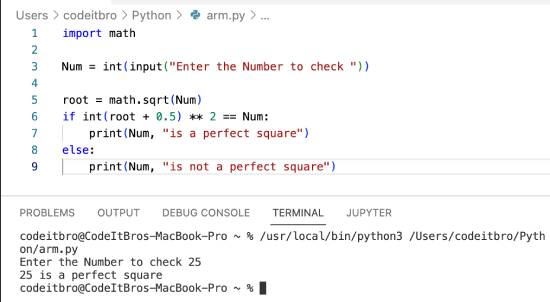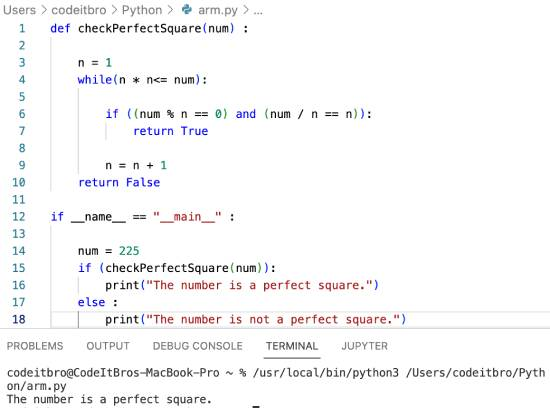# Python Program To Check If A Number Is Perfect Square

22

In this Python program, we will explore how to check if a number is a perfect square or not. A number that is equal to the product of two whole equal numbers is a perfect square. For example, 25 is a perfect square as its square root is 5 – a whole number.

In this tutorial, we will see how to check if a number is perfect in Python using the Sqrt function of the Math library. Apart from that, we will also see how to check if a number is a perfect square or not without using the sqrt function.

## Python Program To Check If A Number Is a Perfect Square Using Sqrt

Algorithm:

Step 1: Take input from the user to check.

Step 2: We will use the the math library’s sqrt function to calculate the user input’s square root.

Step 3: Checking if `int(root + 0.5) ** 2 == Num` is true or not. If this statement is true, then the number is a perfect square; otherwise not.

Code:

```import math

Num = int(input("Enter the Number to check "))

root = math.sqrt(Num)
if int(root + 0.5) ** 2 == Num:
print(Num, "is a perfect square")
else:
print(Num, "is not a perfect square")
```

Output:Explanation:

In the Python program above, we will first import the math library using the `import math`statement. After that, we will take user input and store its value in the Num variable as int.

After that, we calculate the square root of that number using math.sqrt(Num) function and store its value in the root variable.

In the last step, we use Python if-else statements to evaluate if int(root + 0.5) ** 2 is equal to the number or not. If this statement is true, the number is a perfect square, and the statement under the if block is executed.

On the other hand, if the statement is false, then the number is not a perfect square, and the statement under the else block is executed.

## Python Program To Check If A Number Is a Perfect Square Without Sqrt

Code:

```def checkPerfectSquare(num) :

n = 1
while(n * n<= num):

if ((num % n == 0) and (num / n == n)):
return True

n = n + 1
return False

if __name__ == "__main__" :

num = 225
if (checkPerfectSquare(num)):
print("The number is a perfect square.")
else :
print("The number is not a perfect square.")```

Explanation:

In the Python program above, we will run a loop from n = 1 to floor(sqrt(n)) and then check if squaring it makes the num. If it does, then the number is a perfect square otherwise, not.

Output:### Wrapping Up

In this tutorial, we shared how to check if a number is a perfect square or not. In the first method, we used the sqrt() method and in the second one without finding square root.

Other Python tutorials: